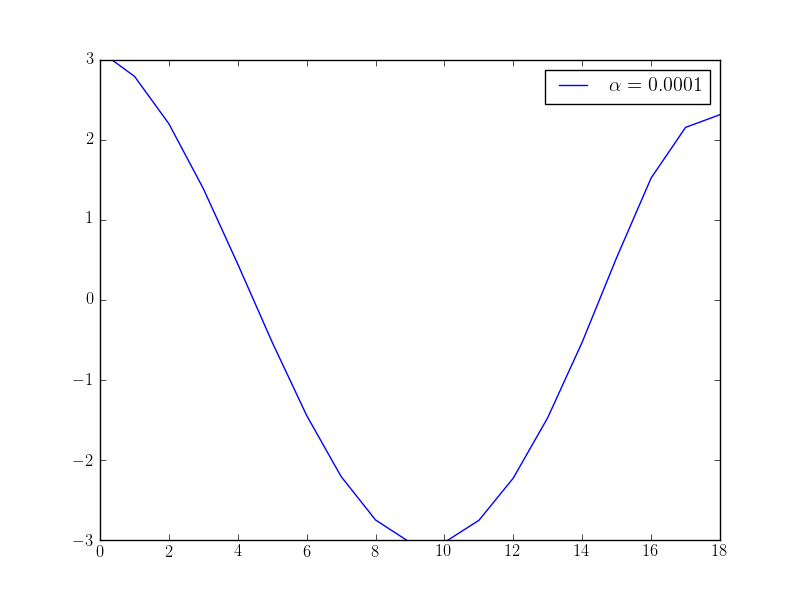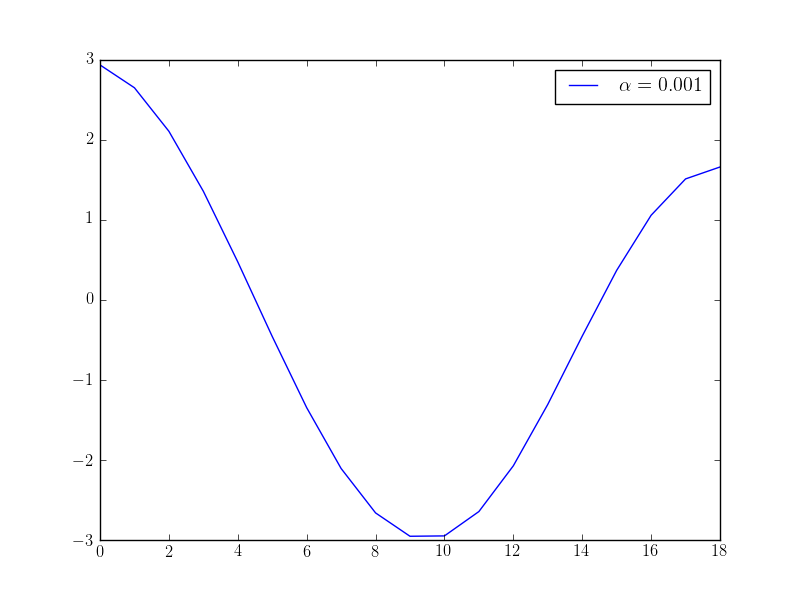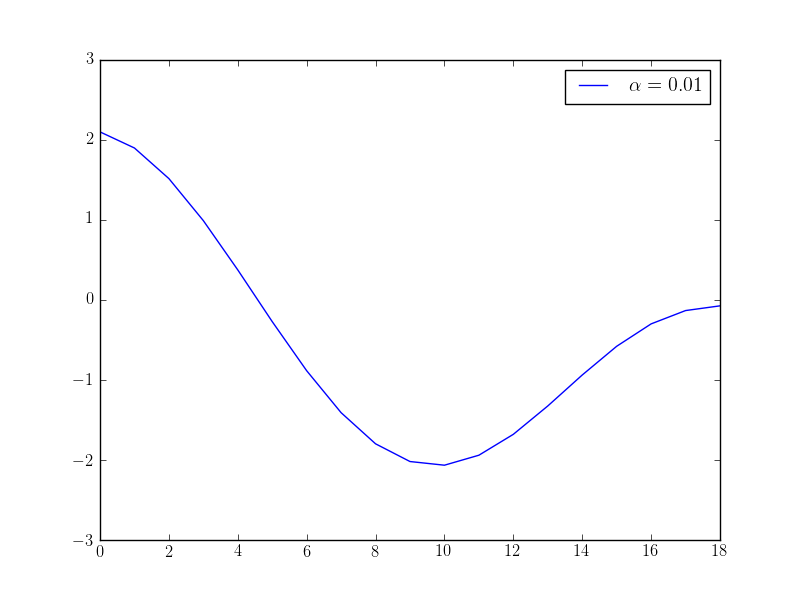# Time-distributed controls¶

Section author: Simon W. Funke <simon@simula.no>

## Background¶

Some time-dependent problems have control variables that are distributed over all (or some) time-levels. The following example demonstrates how this can be implemented in dolfin-adjoint.

One important aspect to consider is the regularisation term. For time-distributed controls, one typically uses wishes to enforce smoothness of the control variables in time. We will also discuss how such a regularisation term is implemented.

## Problem definition¶

We consider the heat equation with a time-dependent source term $$f$$, which will be our control variable:

$\begin{split}\frac{\partial u}{\partial t} - \nu \nabla^{2} u= f(t) \quad & \textrm{in } \Omega \times (0, T), \\ u = 0 \quad & \textrm{for } \Omega \times \{0\} \\ u = 0 \quad & \textrm{for } \partial \Omega \times (0, T).\end{split}$

where $$\Omega$$ is the unit square, $$T$$ is the final time, $$u$$ is the unkown temperature variation, $$\nu$$ is the thermal diffusivity, and $$g$$ is the initial temperature.

The objective value, the model output of interest, is the norm of the temperature variable integrated over time, plus a regularisation term that enforces smoothness of the control in time:

$J(u, f) := \int_0^T \int_\Omega (u-d)^2 \textrm{d} \Omega \text{d}t + \frac{\alpha}{2} \int_0^T \int_\Omega \dot f^2 \textrm{d} \Omega \text{d}t$

The aim of this example is to solve the minimization problem $$\min_f J$$ for some given data $$d$$.

## Implementation¶

We start by importing the needed FEniCS and dolfin-adjoint modules (note that fenics_adjoint is an alias for dolfin_adjoint):

from fenics import *
from collections import OrderedDict


Next, we define the expressions for observational data $$d$$ and the viscosity $$\nu$$.

data = Expression("16*x*(x-1)*x*(x-1)*sin(pi*t)", t=0, degree=4)
nu = Constant(1e-5)


Next, we define the discretization space:

mesh = UnitSquareMesh(8, 8)
V = FunctionSpace(mesh, "CG", 1)


… and time:

dt = Constant(0.1)
T = 2


We are considering a time-distributed forcing as control. In the next step, we create one control function for each timestep in the model, and store all controls in a dictionary that maps timestep to control function:

ctrls = OrderedDict()
t = float(dt)
while t <= T:
ctrls[t] = Function(V)
t += float(dt)


The following function implements a heat equation solver in FEniCS, and constructs the first functional term.

def solve_heat(ctrls):
u = TrialFunction(V)
v = TestFunction(V)

f = Function(V, name="source")
u_0 = Function(V, name="solution")
d = Function(V, name="data")

a, L = lhs(F), rhs(F)
bc = DirichletBC(V, 0, "on_boundary")

t = float(dt)

j = 0.5*float(dt)*assemble((u_0 - d)**2*dx)

while t <= T:
# Update source term from control array
f.assign(ctrls[t])

# Update data function
data.t = t
d.assign(interpolate(data, V))

# Solve PDE
solve(a == L, u_0, bc)

# Implement a trapezoidal rule
if t > T - float(dt):
weight = 0.5
else:
weight = 1

j += weight*float(dt)*assemble((u_0 - d)**2*dx)

# Update time
t += float(dt)

return u_0, d, j

u, d, j = solve_heat(ctrls)


With this preparation steps, we are now ready to define the functional. First we discretise the regularisation term

$\frac{\alpha}{2} \int_0^T \int_\Omega \dot f^2 \textrm{d} \Omega \text{d}t$

Note, that $$f$$ is a piecewise linear function in time over the time intervals $$K = [(0, \delta t), (\delta t, 2 \delta t), \dots, (T-\delta t, T)]$$. Thus, we can write the integral as a sum over all intervals

$\frac{\alpha}{2} \sum_{a_k, b_k \in K} \int_{a_k}^{b_k} \int_\Omega \dot f(t)^2 \textrm{d} \Omega\text{d}t$

Discretising the time-derivative yields:

$\begin{split}\frac{\alpha}{2} \sum_K \int_{a_k}^{b_k} \int_\Omega \left(\frac{f(b_k)- f(a_k)}{b_k-a_k}\right)^2\textrm{d}\Omega \\ = \frac{\alpha}{2} \sum_K (b_k-a_k)^{-1} \int_\Omega \left(f(b_k)- f(a_k)\right)^2\textrm{d}\Omega\end{split}$

In code this is translates to:

alpha = Constant(1e-1)
regularisation = alpha/2*sum([1/dt*(fb-fa)**2*dx for fb, fa in
zip(list(ctrls.values())[1:], list(ctrls.values())[:-1])])


We add the regularisation term to the first functional term and define define the controls:

J = j + assemble(regularisation)
m = [Control(c) for c in ctrls.values()]


Finally, we define the reduced functional and solve the optimisation problem:

rf = ReducedFunctional(J, m)
opt_ctrls = minimize(rf, options={"maxiter": 50})

from matplotlib import pyplot, rc
rc('text', usetex=True)
x = [c((0.5, 0.5)) for c in opt_ctrls]
pyplot.plot(x, label="$\\alpha={}$".format(float(alpha)))
pyplot.ylim([-3, 3])
pyplot.legend()


If we solve this optimisation problem with varying $$\alpha$$ parameters, we observe that we get different behaviour in the controls: the higher the alpha value, the “smoother” the control function becomes. The following plots show the optimised control evaluated at the middle point $$(0.5, 0.5)$$ over time for different $$\alpha$$ values: